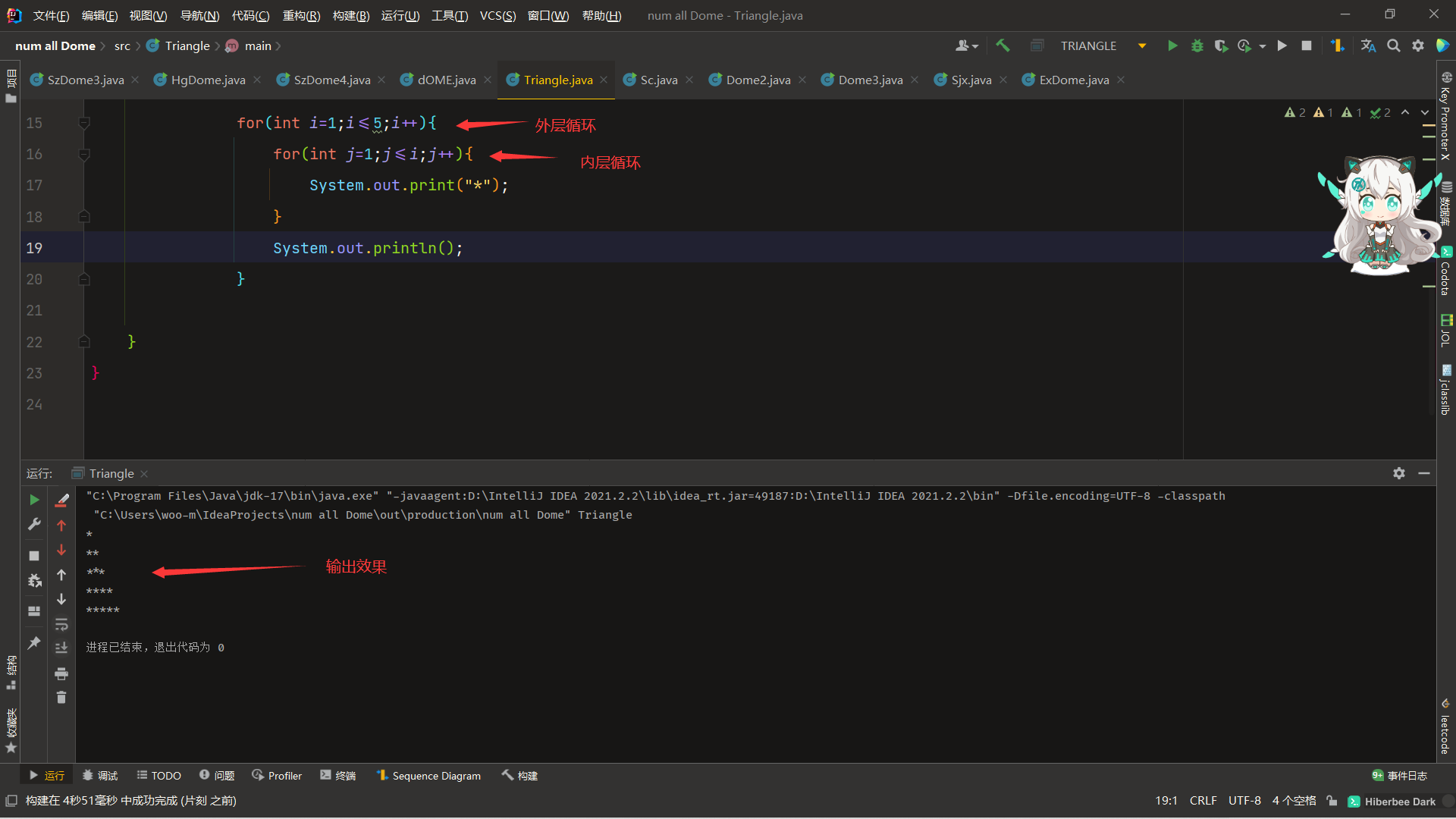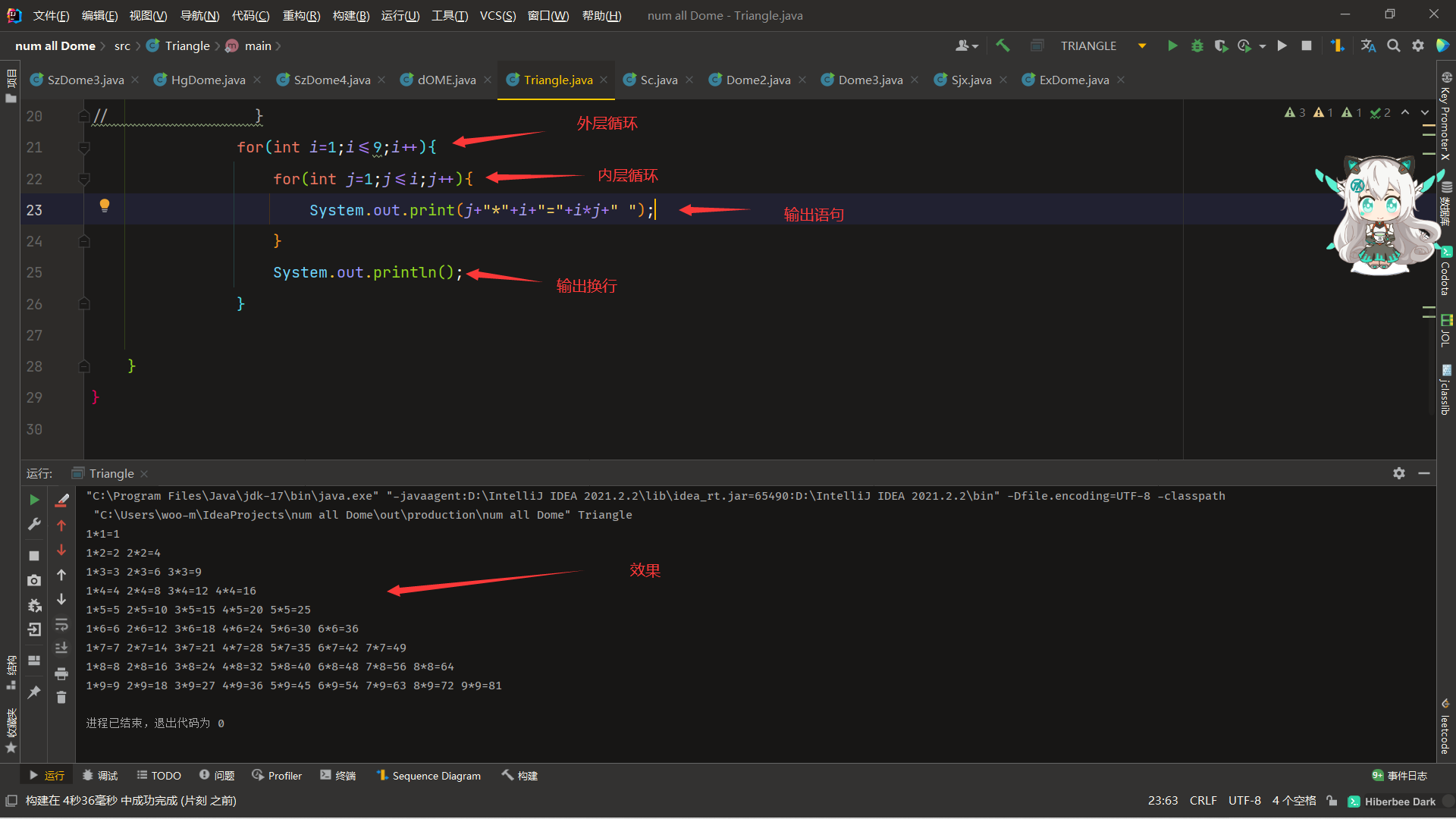• 实例代码 for (int i = 0; i < n; i++) { for (int j = i + 1; j < n; j++) { for (int k = j + 1; k < n; k++) { ...以上代码中，求count++语句的执行次数。 其实这段代码中求count++...

实例代码

for (int i = 0; i < n; i++) {
for (int j = i + 1; j < n; j++) {
for (int k = j + 1; k < n; k++) {
count++;
}
}
}

以上代码中，求count++语句的执行次数。

其实这段代码中求count++语句的执行次数等价于：从n个整数中取出三个整数的组合总共有多少种（不考虑顺序，1,2,3 2,3,1 等算一种）。

求解过程

n &gt; 2 时 ： n&gt;2时：

当 i = 0 时 ， j = 1 ∼ ( n − 1 ) ， c o u n t 的 执 行 次 数 为 ( ( n − 1 ) − 1 ) + ( n − 3 ) + ( n − 4 ) + ⋯ + 2 + 1 当i=0时，j=1\sim (n-1)，count的执行次数为((n-1)-1)+(n-3)+(n-4)+\cdots+2+1

当 i = 1 时 ， j = 2 ∼ ( n − 1 ) ， c o u n t 的 执 行 次 数 为 ( ( n − 1 ) − 2 ) + ( n − 4 ) + ( n − 5 ) ⋯ + 2 + 1 当i=1时，j=2\sim (n-1)，count的执行次数为((n-1)-2)+(n-4)+(n-5)\cdots+2+1

当 i = 2 时 ， j = 3 ∼ ( n − 1 ) ， c o u n t 的 执 行 次 数 为 ( ( n − 1 ) − 3 ) + ( n − 5 ) + ⋯ + 2 + 1 当i=2时，j=3\sim (n-1)，count的执行次数为((n-1)-3)+(n-5)+\cdots+2+1

⋮ \vdots

当 i = n − 3 时 ， j = ( n − 2 ) ∼ ( n − 1 ) ， c o u n t 的 执 行 次 数 为 1 当i=n-3时，j=(n-2)\sim (n-1)，count的执行次数为1

当 i = n − 2 时 ， j = ( n − 1 ) ， c o u n t 的 执 行 次 数 为 0 当i=n-2时，j=(n-1)，count的执行次数为0

可以看出，每次i产生变化时，count++语句的执行次数都是一个等差数列，可由等差数列求和公式：
S n = n ( a 1 + a n ) 2 = 1 + 2 + ⋯ + n = n ( 1 + n ) 2 = n 2 + n 2 S_n=\frac{n(a_1+a_n)}{2}=1+2+\cdots+n=\frac{n(1+n)}{2}=\frac{n^2+n}{2}
count++总执行次数 S n : S_n:
S n = ( n − 2 ) 2 + ( n − 2 ) 2 + ( n − 3 ) 2 + ( n − 3 ) 2 + ⋯ + 2 2 + 2 2 + 1 2 + 1 2 = ( ( n − 2 ) 2 + ( n − 3 ) 2 + ⋯ + 2 2 + 1 2 ) + ( ( n − 2 ) + ( n − 3 ) + ⋯ + 2 + 1 ) 2 S_n=\frac{(n-2)^2+(n-2)}{2}+\frac{(n-3)^2+(n-3)}{2}+\cdots+\frac{2^2+2}{2}+\frac{1^2+1}{2}\\ =\frac{((n-2)^2+(n-3)^2+\cdots+2^2+1^2)+((n-2)+(n-3)+\cdots+2+1)}{2}
再由公式：
1 2 + 2 2 + ⋯ + n 2 = n ( n + 1 ) ( 2 n + 1 ) 6 1^2+2^2+\cdots+n^2=\frac{n(n+1)(2n+1)}{6}
得：
S n = ( n − 2 ) ( n − 2 + 1 ) ( 2 ( n − 2 ) + 1 ) 6 + ( n − 2 ) 2 + ( n − 2 ) 2 2 = n ( n − 1 ) ( n − 2 ) 6 S_n=\frac{\frac{(n-2)(n-2+1)(2(n-2)+1)}{6}+\frac{(n-2)^2+(n-2)}{2}}{2}\\ =\frac{n(n-1)(n-2)}{6}
0 &lt; n &lt; 2 时 ： 0&lt;n&lt;2时：

count++的执行次数为0， n &gt; 2 n&gt;2 的公式仍适用于 0 &lt; n &lt; 2 0&lt;n&lt;2 的情况。

即：

count++语句的执行次数为 n ( n − 1 ) ( n − 2 ) 6 \frac{n(n-1)(n-2)}{6}

参考文章

一个小问题：计算3层循环的执行次数

展开全文• 双重for循环（嵌套for循环）

千次阅读 多人点赞 2021-10-13 16:20:32
什么是双重循环双重循环：我们从字面上理解它，就是有两个循环套在一起 详细解释 双重for循环就是在一个for循环里在嵌套另一个for循环，即两个循环嵌套，还有多重循环嵌套的情况，但用的比较少，一般常用的是...

什么是双重循环？

双重循环：我们从字面上理解它，就是有两个循环套在一起

详细解释

双重for循环就是在一个for循环嵌套另一个for循环，即两个循环嵌套，还有多重循环嵌套的情况，但用的比较少，一般常用的是两个循环嵌套，很少使用多重循环，因为for循环多了会导致代码执行的效率低，而且容易死机,多循环中的总循环次数是相乘的，嵌套几次后几何级数增长，因此通常情况下在循环多次嵌套时，我们是不推荐使用的。

说的再简单点就是，for循环的嵌套，跟我们之前的if嵌套一样

for(循环变量;循环条件;循环操作){
for(循环变量;循环条件;循环操作){
//代码块
}
}

这就是双重for循环的框架

运行路径

外层循环一次内层全部循环一次（外层循环一次，内层for循环全部执行完成再进行外层的一次循环）

难点

二重循环的难点是内层循环的循环条件，如何控制内层循环的次数
记住外层循环一次，内层循环全部的原则
举个例子，我外层循环定义循环3次内层循环定义循环2次，每次输出一个“星号”，那么，将会输出几个“星号”呢？
答案是6次（2×3）
分析:

• 外层循环第一次，里面循环完，也就是2次
• 外层循环第二次，里面又重新循环，循环完，还是2次
• 外层循环第三次，里面再次重新循环，又是2次

我们多说无益，来个实际操作

示例

打印以下图形，我们可以用上面提到的公式验证一下

图形

*
* *
* * *
* * * *
* * * * *

这个题目的代码，我先给出，然后分析

代码

public class Star{
public static void main(String[] args){
for(int i=1;i<=5;i++){
for(int j=1;j<=i;j++){
System.out.print("*");
}
System.out.println();
}
}
}

我们看看这个图像有多少行？ 总共有5行，也就是说我们需要循环5次打印才可以

外部循环

for(int i=1;i<=5;i++){
//循环块2
}

这个循环控制输出的行数，我们这里是5行“星号”，所以这个循环控制成5次

我们开始写里面的循环

内部循环

里面定义一个循环，变量为 j

for(int j=1;j<=i;j++){
System.out.print("*");
}

的初始值定义为1，并且j<=i （i也是累加，到5停止），它的循环次数也是循环5次，因为我们这个图像的最后一个输出是5颗“星号”，这里是循环输出了5颗“星号”，所以，j超出5必须停止循环

总体运行过程

到这，许多人会感到有点迷茫，不过没有关系，这是正常，我们好好看看它如何运行：

首先注意看变量：i=1、j=1、i<=5、j

循环运行变化

外层第一次循环： i为1时，开始第一次循环，开始运行里面的第 i（1）次循环，这时，里面的循环，j为1时j<=i 此时i为1），所以会输出1颗“星号”

拆解代码：第一次外层循环
内层

for(int j=1;j<=1;j++){
System.out.print("*");
}
System.out.println();

外层第三次、第四次、第五次与第二次一样i不断累加，依次输出3颗“星号”、4颗“星号”、5颗“星号”

就不多演示了，还不懂的话，就看看拆解代码的内层循环的循环条件的变化注意：System.out.print（"*"）:没有ln的，所以不会换行，于是内层循环的“星号”是横排叠加的

示例二

打印九九乘法表
因为这个题目，和上面的代码以及原理一样，只是改变了一些内容，所以我就直接放代码了

代码

public class Jjcfb {
public static void main(String[] args) {
for(int i=1;i<=9;i++){
for(int j=1;j<=i;j++){
System.out.print(j+"*"+i+"="+i*j+" ");
}
System.out.println();
}
}
}

九九乘法表一共有九行，所以外层限制行数为9，于是i=1;i<=9
内层还是j=1;j<=i
如果还不懂，可以用IDEA调试慢慢摸索，这样记忆力更加深刻扩展

给大家放一个问题：这样用“*”输出一个菱形

展开全文java 循环 for循环 嵌套 算法
• 双重for循环嵌套执行原理

千次阅读 2020-03-21 22:15:25
双重for循环嵌套 先简单介绍下单个for循环的原理： for(定义变量;循环条件;变量变化){ 循环语句块; } 1、定义变量在进入循环执行一次； 2、循环条件是每次进入循环之前都会执行并判断； 3、变量变化是每次...

双重for循环嵌套

先简单介绍下单个for循环的原理：

for(定义变量;循环条件;变量变化){
循环语句块;
}
1、定义变量在进入循环前执行一次；
2、循环条件是每次进入循环之前都会执行并判断；
3、变量变化是每次完成循环语句块后执行的内容；

下面看一段代码：

// 逆战 停课不停学 加油！！

for (i = 1; i < 5; i++) {
for (j = 1; j < 5; j++) {
}
}

当执行程序时，先执行外层 i 的循环;
当 i 第一次循环时 i = 1，满足条件 i < 5，所以进入 i 的循环语句块（也就是 j 循环）；当 j 第一次循环式 j =，满足条件 j <5，所以进入 j 的循环语句块；为了方便理解，我们把代码稍微变动如下：

// 逆战 停课不停学 加油！！

for (i = 1; i < 5; i++) {
document.write("外层第" + i + "次循环<br><br>");
for (j = 1; j < 5; j++) {
document.write("内层第" + j + "次循环<br>");
}
document.write("<br>");
}

效果图：此时 j 第一次循环，就会执行
document.write("内层第" + j + "次循环<br>");
然后 j++，继续进行判断循 j 的环条件是否为true，如果条件为true则继续执行循环语句块，直到循环条件为false时停止循环；
继续执行 i 循环；
document.write("<br>");
然后 i++，

当 i 第二次循环时，i = 2 ，满足条件 i < 5，继续进入 i 循环语句块，也就是继续循环一次 j 循环，也就是说 i 每次循环时，j都会重新执行一个完整的循环，这样就形成了内外层的循环嵌套。

展开全文javascript
• 双重for循环

千次阅读 2020-12-14 18:51:46
当i=1时，执行内层循环从j=1到j=i，内层结束后开始 i++, 当i=2时，执行内层循环从j=1到j=i，内层结束后开始 i++, … 当i=9时，执行内层循环从j=1到j=i，内层结束后开始 i++,此时i=10；外层循环结束 for(var i=1;i<...

什么是双重for循环

双重for循环就是在一个for循环里在嵌套另一个for循环，ji\即两个循环嵌套，还有多重循环嵌套的情况，但用的比较少，一般常用的是两个循环嵌套，很少使用多重循环，因为for循环多了会导致代码执行的效率低，而且容易死机,多循环中的总循环次数是相乘的，嵌套几次后几何级数增长，因此通常情况下在循环多次嵌套时，我们是不推荐使用使用的。

语法

外层循环一次，内层for循环全部执行完成再进行外层的一次循环；

for(循环变量;循环条件;循环操作){
for(循环变量;循环条件;循环操作){
}
}

打印99乘法表

当i=1时，执行内层循环从j=1到j=i，内层结束后开始 i++,
当i=2时，执行内层循环从j=1到j=i，内层结束后开始 i++,

当i=9时，执行内层循环从j=1到j=i，内层结束后开始 i++,此时i=10；外层循环结束

for(var i=1;i<=9;i++){
for(var j=1;j<=i;j++){
document.write(i+"*"+j+"="+Number(i*j)+"\t"+"&nbsp;");  //\t为制表符  &nbsp为空格
}
document.write("<br/>")  //换行
}打印倒三角

当i=1时，开始内层循环，j从8开始到j=i,打印*，内层结束后开始i++;

当i=8时，再次执行内层循环，j从8开始到 j=1,内层循环结束后i++,此时i=10;循环完毕

for(var i=1;i<=8;i++){
for(var j=8;j>=i;j--){
document.write("*");
}
document.write("</br>"); //打印换行
}打印正三角

原理与打印倒三角相似， 只是内层循环有些不同；

for(var i=1;i<=5;i++){
for(var j=1;j<=i;j++){
document.write("*");
}
document.write("</br>");
}

结果：展开全文• 首先，我们说一下循环嵌套的执行原理。 字面意思，循环嵌套就是循环之中，循环体也有另一个循环。也就是 for(){} 的循环体,也...外层循环执行一次,内层循环执行所有。 for(){ for(){} } 执行程序,先执行外层 j ...javascript
• IE没有我们想象中笨，它知道总的循环次数还是一千万次。因此，得把这一百个十万次循环分开执行。虽然Javascript是单线程的，但也可以通过setTimeout或setInterval模拟多线程。
• JavaScript for循环 双重循环

千次阅读 2020-09-21 16:32:21
for循环 语法结构 for(初始化变量;条件表达式;操作表达式){ //循环体 } 初始化变量: 通常被用于初始化一个计数器，该表达式可以使用var关键字声明新的变量...执行条件表达式，如果为true，则执行循环体语句，否则退出
• 循环次数较少的时候for循环一般不会影响运行效率，但一旦循环次数较多，成千上万的时候，循环次数越多，效率就越慢，最近在做一程序的时候要解决优化问题，反复查看只有优化双重for循环才能提高效率。事实证明，优化...
• for循环-双重for循环

千次阅读 2020-11-30 08:50:44
for循环-双重for循环 1 - 循环 1.1 for循环 语法结构 for(初始化变量; 条件表达式; 操作表达式 ){ //循环体 } | 名称 | 作用 | 初始化变量 | 通常被用于初始化一个计数器，该表达式可以使用 var 关键字声明...
• C语言概述C语言的执行速度快，执行效率高，功能强大，编程自由。 但缺点也是有的，代码实现的周期较长，可移植性较差， 由于过于自由，模块的操作单一(历史性原因)。 因为C语言是底层代码，所以需要了解计算机的基本...
• 冒泡（起泡）排序网上一搜有很多，我这里只是记录下我的感想： 冒泡排序里有这么一段： ...这要从冒泡排序原理说起：冒泡排序每循环一次,就把最大的数排在了最右边（不信你试试），所以下次循...
• for循环: 初始化 变量： 通常被用于初始化一个计数器，该表达式可以使用 var 关键字声明新的变量，这 个变量帮... 执行条件表达式，如果为true，则执行循环体语句，否则退出循环循环结束。 1. 执行操作表达式，此时js
•算法 数据结构 c语言
• 7.1 for循环 ​ for ( 初始化变量;...操作表达式：会在循环（代码块）每次被执行执行。是每次循环最后执行的代码 经常用于我们计数器变量进行更新（递增或者递减） for(var i = 0; i<=5; i++ ){ console.
• shell之for、while、until循环shell编程循环语句for语句应用示例while语句结构while小实验until语句 shell编程循环语句 读取不同的变量值，用来逐个执行同一组命令 格式： for 变量名 in 聚会列表 do 命令序列 ...
• 1、复习循环控制结构,学习内容,3、while循环,5、for循环,6、循环的嵌套,4、do-while循环,7、几种循环结构的比较,1、循环控制概述,2、goto语句和goto循环,重难点,1、 while语句、do-while语句和for语句及三种循环之间...
• 1、循环 1、双重for循环 **概述：**循环嵌套是指在一个循环语句中再定义一个循环语法...外层循环执行一次，内层循环要执行完有的次数 外层循环控制行数，内层循环控制每行个数 循环的总个数=外层循环的次数*内层循环的js
• 在阅读这篇文章是，需要一定的基础，如题目所示，要知道会用C++ FOR单循环语句，否则将很难理解双循环。 因为在主流的语言中，大部分的语言for循环语句没有多大区别，因此，这里用C++来做简单的演示（其实C也可以）...c++ html c语言
• 适用于 区间判断 | 确定循环次数 的场合 执行流程: 1.条件初始化 2.条件判断 3.不满足条件结束循环;满足条件则执行循环体语句体 4条件变量 注意: 条件初始化只执行一次 语法结构: for (条件初始化; 条件判断 ; ...for while
• python中双循环

千次阅读 2020-11-30 11:00:13
如果你对python中的for循环不是很清楚，请看看这篇文章：for循环控制语句——菜鸟的python笔记“下面是我做的一些学习记录供大家参考：1 #基本的for循环语句 2 test_list = 3 #打印列表的长度 4 print(len(test_list...
• 总结：这里面最关键的是要搞懂，外层for循环控制行数，内层for循环控制个数，然后要明白这种for循环的运行机制，要知道外层循环一次，里面的for循环要把该循环次数循环完成，才再次进行外层for循环的第二次javascript js
• 目录1、靠左的直角三角形2、靠右的直角三角形3、等腰三角形4、平行四边形5、...外循环次数决定内循环执行遍数。 1、靠左的直角三角形 #!/bin/bash\ for ((a=1; a<=9; a++)) #9行 do for ((b=1; b<=\$a; b++))
• 双重循环，先用栈保存cx mov si,0 mov cx,4 s:mov al,[bx+3+si] ;从第四个字母开始，用si来依次推进 and al,11011111b ;变为大写字母 mov [bx+3+si],al inc si loop s add bx,16 ;每次循环结束...编程语言
• 主要学习内容：Math用法三角函数指数函数方法取整方法min,max,abs,random方法循环控制语句for循环while循环while语句do...while语句跳出循环关键字breeak关键字continue关键字双重循环数组数组的定义数组的特点数组...循环语句
• 今天用Python写程序的时候，写了两个for循环，结果发现循环次数没有达到预期（也就是两个循环次数相乘的循环次数）   解决方法 通过调试发现，当外循环进行第一次的时候，内循环正常工作，可是，当外循环...Python for 循环 生成器
• 在上一篇文章中提到了程序逻辑通过顺序、分支、循环三种结构来实现的，并介绍了分支结构的执行语句，那么今天这篇文章介绍实现程序逻辑的另一种结构——循环结构。什么是循环结构循环结构是指在程序设计语言中按照...
• 循环嵌套是指在一个循环语句中再定义一个循环语句的语法结构，例如在for循环语句中，可以再 嵌套一个for 循环，这样的 for 循环语句我们称之为双重for循环双重 for 循环语法 for (外循环的初始; 外循环的条件;...蓝桥杯
• for循环 for循环就是对数组的元素进行循环。 语法： for (初始化变量; 条件表达式; 迭代语句) { 需要执行的代码块；...for循环执行顺序： // 1. 声明变量； // 2. 判断循环执行条件； // 3. 代码块javascript
•javascript
• 有时候项目中会出现双重for循环校验，类似下面这种：for (int i = 0; i ; i++) { // 外循环 for (int j = i + 1; j ; j++) { // 内循环 //校验规则。。。 } }像这种，外循环的每个i值都要和内循环的i之后的所有...多线程 优化...